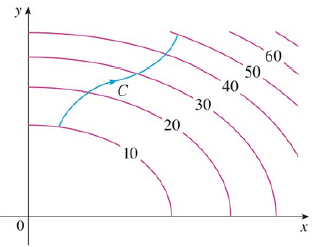Chapter 16.3, Problem 1E

Chapter
Section
Textbook Problem

The figure shows a curve C and a contour map of a function f whose gradient is continuous. Find ∫C ∇ f · dr.To determine

To find: The value of Cfdr .

Explanation

Definition:

Refer to FIGURE 1 of section 16.3 in the textbook.

Consider a smooth curve represented by a vector function r(t) , atb .

If f is the differentiable function of two or three variables with gradient vector f is continuous on curve C then Cfdr ,

Cfdr=f(r(b))f(r(a)) (1)

Re-modify given Figure as shown in Figure 1

Still sussing out bartleby?

Check out a sample textbook solution.

See a sample solution

The Solution to Your Study Problems

Bartleby provides explanations to thousands of textbook problems written by our experts, many with advanced degrees!

Get Started

Define a Type I error and a Type Il error and explain the consequences of each.

Statistics for The Behavioral Sciences (MindTap Course List)

find the real roots of each equation by factoring. 129. x2 + x 12 = 0

Applied Calculus for the Managerial, Life, and Social Sciences: A Brief Approach

True or False: The Alternating Series Test may be applied to determine the convergence of n=12+(1)n2n2.

Study Guide for Stewart's Single Variable Calculus: Early Transcendentals, 8th

45 15 63 127

Study Guide for Stewart's Multivariable Calculus, 8th